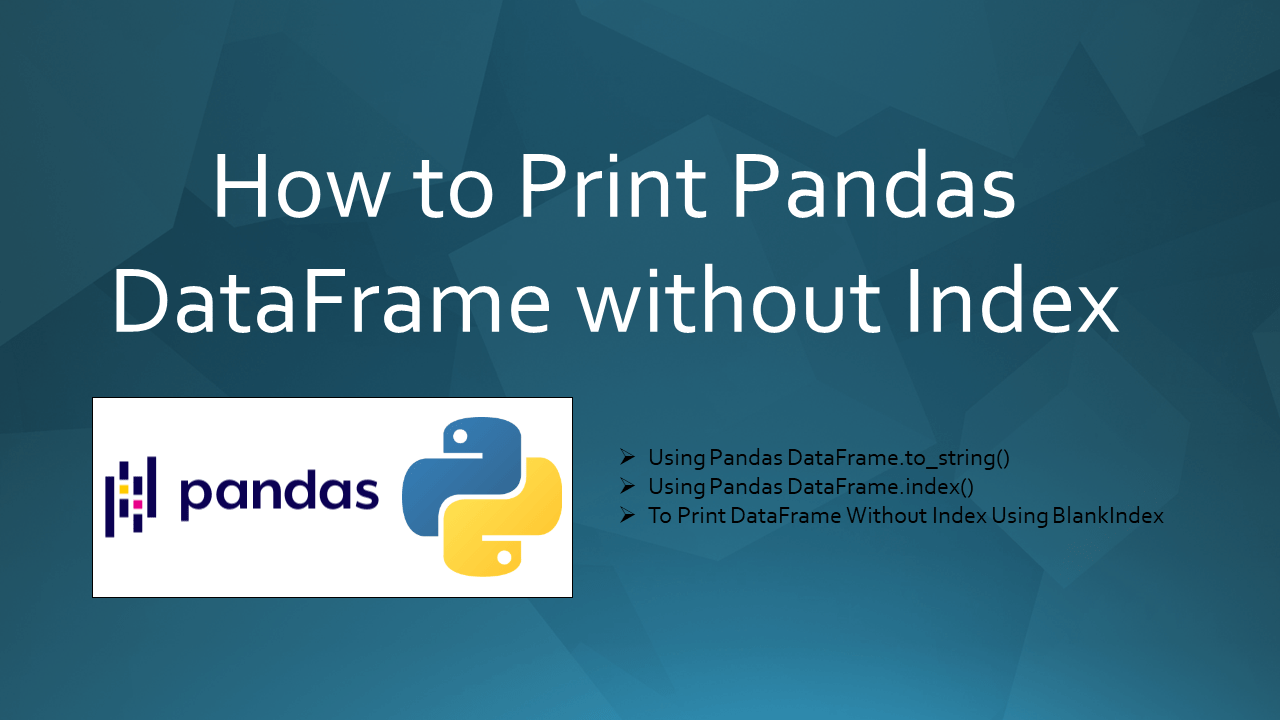# How to Print Pandas DataFrame without Index

• Post author:
• Post category:Pandas

To print the Pandas DataFrame without an index you can use `DataFrame.to_string()` and set the index parameter as False. A Pandas DataFrame is a powerful data structure that consists of rows and columns, each identified by their respective row index and column names. When you print a DataFrame, the row index is typically displayed as the first column. In this article, I will explain how to print pandas DataFrame without index with examples.

## 1. Quick Examples of Print Pandas DataFrame without Index

If you are in a hurry, below are some quick examples of how to print Pandas DataFrame without an index.

``````
# Below are quick example

# Example 1: Using DataFrame.to_string() to print without index
df2 = df.to_string(index=False)

# Example 2: Using BlankIndex to print DataFrame without index
blankIndex=[''] * len(df)
df.index=blankIndex

# Example 3: Using hide_index()
df.style.hide_index()
``````

Now, let’s create a DataFrame with a few rows and columns, execute these examples, and validate the results. Our DataFrame contains column names `Courses`, `Fee`, `Duration`, and `Discount`.

``````
# Create DataFrame
import pandas as pd
technologies = {
'Courses':["Spark","PySpark","Python","pandas"],
'Fee' :[20000,25000,22000,30000],
'Duration':['30days','40days','35days','50days'],
'Discount':[1000,2300,1200,2000]
}
index_labels=['r1','r2','r3','r4']
df = pd.DataFrame(technologies,index=index_labels)
print("Create DataFrame:\n", df)
``````

Yields below output.

## 2. Using DataFrame.to_string() to Print DataFrame without Index

You can use `DataFrame.to_string(index=False)` on the DataFrame object to print the DataFrame without an index. To result `DataFrame.to_string()` function is a string of the DataFrame without indices. The column names are retained as the first row.

``````
# Using DataFrame.to_string() to print without index
df2 = df.to_string(index=False)
print("Get the DataFrame without an index:\n", df2)
``````

Yields below output.

Alternatively, you can also use to_string() method to print the DataFrame of specified columns without an index.

``````
# Using DataFrame.to_string() to print without index
# of specified columns
specified_col = ['Courses', 'Discount']
df2 = df[specified_col].to_string(index=False)
print("Get the DataFrame without an index:\n", df2)
``````

Yields below output.

``````
# Output:
Get the DataFrame without an index:
Courses  Discount
Spark      1000
PySpark      2300
Python      1200
pandas      2000
``````

## 3. To Print DataFrame Without Index By Making Index empty

You can set the index as empty for each row, you can do this by creating an array with the empty string (one for each row in the DataFrame). and assign this to the `DataFrame.inde`x property.

``````
# Print DataFrame without index
blankIndex=[''] * len(df)
df.index=blankIndex
print("Get the DataFrame without an index:\n", df)
``````

Yields below output.

``````
# Output.
Get the DataFrame without an index:
Courses    Fee Duration  Discount
Spark  20000   30days      1000
PySpark  25000   40days      2300
Python  22000   35days      1200
pandas  30000   50days      2000
``````

## 4. Use hide_index()

By using `hide_index()` method, you can hide the Index of the DataFrame. This is one of the best approach if you are using Python 3.7 or the latest.

``````
# Using hide Index
df.style.hide_index()
print("Get the DataFrame without an index:\n", df)
``````

Yields the same output as above.

## 5. Print DataFrame without Index on Jupyter Notebook

These days many developers and data analysts use Jupyter Notebook to run the Pandas, so to remove and print the DataFrame without index use `display()` method.

``````
# Display without index on Jupyter.
display(df.hide_index())
``````

## 6. Write to CSV without an Index

In case, if you want to write a pandas DataFrame to a CSV file without an Index, use the param `index=False` in `to_csv()` method.

``````
# Write CSV file by ignoring Index.
print(df.to_csv(index=False))
``````

If you want to select some columns and ignore the index column.

``````
print(df.to_csv(columns=['Fee', 'Duration'], sep='\t', index=False))
``````

## 7. With Python 2.7

With Python 2.7, print has a different syntax. Use the below to print DataFrame in the Python 2.7 version.

``````
# With older Python version
print df.to_string(index=False)
``````

Yields the same output as above.

## 8. Complete Example For Print DataFrame without Index

``````
# Example For Print DataFrame without Index
import pandas as pd
technologies = {
'Courses':["Spark","PySpark","Python","pandas"],
'Fee' :[20000,25000,22000,30000],
'Duration':['30days','40days','35days','50days'],
'Discount':[1000,2300,1200,2000]
}
index_labels=['r1','r2','r3','r4']
df = pd.DataFrame(technologies,index=index_labels)
print(df)

# Using DataFrame.to_string() to print without index
df2 = df.to_string(index=False)
print(df2)

# Using BlankIndex to print DataFrame without index
blankIndex=[''] * len(df)
df.index=blankIndex
print(df)
``````

## Frequently Asked Questions of Print Pandas DataFrame without Index

How to print a Pandas DataFrame without the index using to_string()?

You can print the DataFrame without an index using the `to_string()` method and set the `index` parameter to `False`. For example, `df.to_string(index=False)`

How to display a Pandas DataFrame without the index using to_string() with specific columns?

You can call the to_string(index = False) method with specified columns of DataFrame to display the DataFrame without an index with specified columns. For example, `df[specified-columns].to-string(index=False)`

How to output a Pandas DataFrame to a CSV file without including the index?

You can write a pandas DataFrame to a CSV file without an Index, using the param `index=False` in `to_csv()` method.For example, `df.to_csv( index=False)`

How to print a Pandas DataFrame without the index in a Jupyter Notebook?

You can use Jupyter Notebook to run the Pandas, so to remove and print the DataFrame without index use `display()` method. For example, `display(df.hide_index())`

## Conclusion

In this article, you have learned how to print pandas DataFrame without an index or ignore an index using `to_string()`, `index()` and `hide_index()` functions with examples.

Happy Learning !!

## References

### Naveen

I am a Data Engineer with 20+ years of experience in transforming data into actionable insights. Over the years, I have honed my expertise in designing, implementing, and maintaining data pipelines with frameworks like Apache Spark, PySpark, Pandas, R, Hive and Machine Learning. My journey in the field of data engineering has been a continuous learning, innovation, and a strong commitment to data integrity. I have started this SparkByExamples.com to share my experiences with the data as I come across. You can learn more about me at LinkedIn